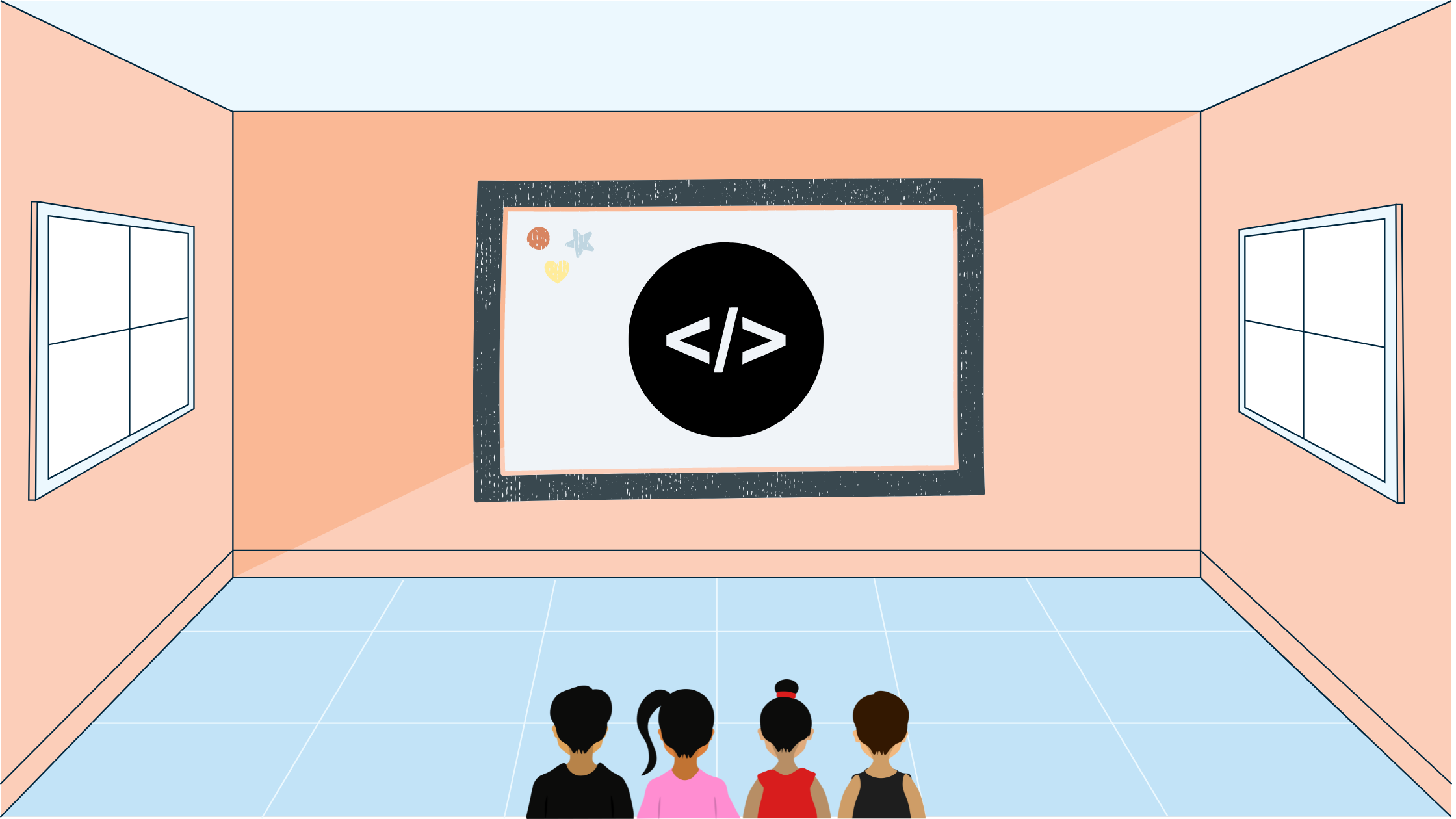Yash Singh# USACO Photoshoot II

In this blog post I'll go over the USACO Photoshoot II Bronze February 2022 Problem 2 using other resources and solutions.

## Statement

We are given two arrays of integers that are contain the numbers from 1 through $n$ rearranged. Our job is to find the minimum number of operations to get from array one to array two. One operation consists of moving an integer to the left in the array.

## Solution

Instead of trying to get from array one to array two, let's convert the numbers so array two is just one through $n$ consecutively and array one is respectively rearranged. For example:

5 3 4 2 1 --> 5 1 4 2 3
3 2 1 4 5 --> 1 2 3 4 5

This way we can know what position we have to shift to relative to the index that we are iterating on in the array. We can maintain a max and whenever anything appears underneath it while we are iterating it, we will increment an operations counter.

## Implementation

Let's go through an implementation in C++:

#include <bits/stdc++.h>

using namespace std;

int main() {
ios_base::sync_with_stdio(false);
cin.tie(nullptr);
int n;
cin >> n;
map<int, int> m;
int a[n];
for (int i{0}; i < n; ++i) {
cin >> a[i];
}
// store value -> index in m and index -> value in a
for (int i{0}; i < n; ++i) {
int x;
cin >> x;
m[x] = i;
}
int mx{-1};
int ans{0};
// iterate through first array
// if current element is a max, then we don't need
// to shift it behind another element
// or else increment the answer
for (int i{0}; i < n; ++i) {
if (m[a[i]] > mx) {
mx = m[a[i]];
} else {
++ans;
}
}
cout << ans;
}

## Lessons Learnt

A lesson learned in this problem is to find out some way to simplify the input. We had two different arrays from which we had to find a connection, but instead we made it so that the presence of the second array wasn't even needed. Also, try testing out different inputs with a pen and paper. This can help bring about key observations and patterns.